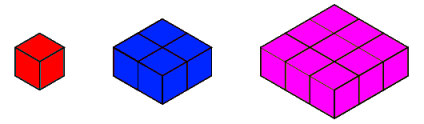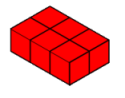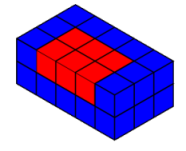#### You may also like### Just Rolling Round

P is a point on the circumference of a circle radius r which rolls, without slipping, inside a circle of radius 2r. What is the locus of P?### Coke Machine

The coke machine in college takes 50 pence pieces. It also takes a certain foreign coin of traditional design...### Just Opposite

A and C are the opposite vertices of a square ABCD, and have coordinates (a,b) and (c,d), respectively. What are the coordinates of the vertices B and D? What is the area of the square?

# Summing Squares

##### Age 14 to 16Challenge Level

You may wish to make six copies of each square prism below to aid you in visualising the problem that follows.If I take six of the red cubes I can make this 3 by 2 by 1 cuboid:I want to surround this with blue cubes to make the 5 by 3 by 2 cuboid below:Could I build this cuboid by adding on six blue square prisms like the one above without splitting any of them?
If so, describe how to do it. If not, explain why not, and describe how to arrange the blue square prisms so that as few as possible need to be split.
I want to surround this with pink cubes to make a 7 by 4 by 3 cuboid. (See Hint for a picture of this cuboid.)
Could I build it by adding on six pink square prisms like the one above? Do I need to split any this time?

So far, we've discovered that
$$6 \times 1^2 = 3 \times2 \times1$$
$$6\times(1^2+2^2) = 5\times3\times2$$
$$6\times(1^2+2^2+3^2) = 7\times4\times3$$

Can you describe a method for forming the next cuboid in the sequence by adding on six square prisms? You will only need to split one of your square prisms each time. Does the sequence continue forever?

Work out the dimensions of the $n^{th}$ cuboid.
Can you explain how to make the $(n+1)^{th}$ cuboid from it? What are the dimensions of the $(n+1)^{th}$ cuboid?

How could these ideas be used to calculate the sum $1^2+2^2+3^2+...+n^2$ quickly for any value of $n$?